# Power input

The construction lift lifted a load weighing 300 kg to a height of 12 m with a uniform movement. How long has it taken for the load to be lifted if the efficiency is 75% and the engine has a power input of 5 kW?

t =  9.6 s

### Step-by-step explanation: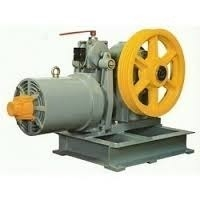Did you find an error or inaccuracy? Feel free to write us. Thank you!Tips to related online calculators
Do you want to convert mass units?
Do you want to convert time units like minutes to seconds?

## Related math problems and questions:

• Elevator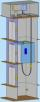In homes with more floor elevators are used. For passenger transport, the most commonly used traction elevator counterweight. The top of the shaft engine room with the engine. The car is suspended on a rope, which is guided up over two pulleys to the coun
• Electric cooker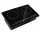During which time t does an electric cooker with power input P = 500 W and with efficiency n = 75% heat water with mass m = 2 kg and temperature t1 = 10°C to the boiling point (t2 = 100°C). The specific heat capacity of water is c = 4 180 J. Kg-1. K-1
• Efficiency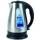What is the power output of a kettle 2 kW with an efficiency of 90%?
• Motorcycle engineThe motorcycle engine has a constant power of 1.2 kW for 0.5 hours. How much work does the engine do? Express work in kWh and Ws units.
• WeightlifterWeightlifter lifted 75% of its weight. Determine how much weight lifted when he weighs 132 kg.
• Orlík hydroelectric plantThe Orlík hydroelectric power plant, built in 1954-1961, consists of four Kaplan turbines. For each of them, the water with a flow rate of Q = 150 m3/s is supplied with a flow rate of h = 70.5 m at full power. a) What is the total installed power of the p
• Concrete columnThe concrete column of the highway bridge has the shape of a block with dimensions of 1m x 0.8m x 25m. It should be lifted by crane to a height of 20m. What is the power of his engine if the lifting takes 2 minutes?
• Barrel 3Barrel with water weights 118 kg. When we get off 75% of water, it weights 35 kg. How many kg has an empty barrel?
• The water barrel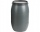The water barrel weighs 122 kg. If we pour 75% of the water out of it, it will weigh 35 kg. What is the weight of the barrel?
• Free fall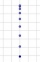The elevator car weighing 720 kg is torn off at a height of 125 m and begins to fall in a free fall. How much kinetic energy does the elevator car have when it hits the ground floor of the building, when the free fall of the car lasted 5 s?
• CarAt what horizontal distance reaches the car weight m = 753 kg speed v = 74 km/h when the car engine develops a tensile force F = 3061 N. (Neglect resistance of the environment.)
• Inclined plane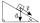1. How much work W we have to do to pull a body weighing 200 kg along an inclined plane with a length of 4 m to a total height of 1.5 m. 2. Find the force we need to exert to do this if we neglect frictional resistance. 3. Find the force we would need if
• Friction coefficientWhat is the weight of a car when it moves on a horizontal road at a speed of v = 50 km/h at engine power P = 7 kW? The friction coefficient is 0.07
• Positional energyWhat velocity in km/h must a body weighing 60 kg have for its kinetic energy to be the same as its positional energy at the height 50 m?
• Sand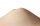How much m ^ 3 of sand can be loaded on a car with a load capacity of 5 t? The sand density is 1600 kg/m3 .
• Container with water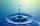The weight of a container with water is 2.48 kg. If the cast 75% water, the container with water has a weight of 0.98 kg. Determine the weight of the empty container. How much water was originally in the container?
• Motor physics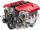A car is traveling at 98 km/h on the highway. The pulling power of the motor is 6 kN. Find the engine power in kW.